# Using the Aggregate Operator

## Introduction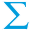Use the Aggregate operator to compute aggregations of tuple values over moving windows that partition its input. Each window is a view on a part of the input data. For example, you can define aggregations that sum the values of a data field over 30-second intervals; a window opens, admits tuples for 30 seconds, closes and emits a field containing the sum. If you define windows that overlap in time, an Aggregate operator can calculate moving averages of any time-varying quantity.

The Aggregate operator accepts a single input stream. It maintains the state of one or more windows according to policies defined in the operator's properties. The principal policies define the conditions under which aggregate windows open (begin accumulating state), close (stop accumulating state), and emit results (calculations over the accumulated state). You define window policies in one or more dimensions. A dimension specifies:

• How windows are to be anchored on the input data, the dimension value, which can be based on wall clock time, tuple counts, values in the stream, or arbitrary predicates.

• When a window closes. For example, a window might close when it contains some number of tuples or when some number of seconds elapse.

• When a window opens, in terms of how much each new window advances relative to the previous one. For example, a window might open every 5 minutes.

• When the window emits. Windows emit tuples when they close, but can also emit tuples while they are open. For example, a window might emit every time it receives five tuples.

• How to handle boundary conditions, such as windows which would have opened before the first input is received, or windows which occur during a gap in the data.

Using the Edit Dimension dialog, you can define dimensions in four different ways:

• Tuple dimension — Window policies are based on the number of tuples occupying a window.

• Field dimension — Window policies are based on (non-decreasing) values in input fields.

• Time dimension — Window policies are based on elapsed units of time, usually seconds.

• Predicate dimension — Window policies are based on evaluating predicate expressions.

Although a dimension collects specific Open, Emit, and Close expressions into a single named configuration, these events act independently on all available windows, resulting in windows opened by one dimension being examined for Emit and Close by another dimension.

Each dimension's Open expression is evaluated regardless of the result from other dimensions. This is reasonable and expected, but the case where the Open expressions are identical for two or more dimensions results in identical windows opening which is redundant. The expectation is that the Open expressions are different and result in unique sets of windows without perfect duplication.

When the conditions prescribed in the dimension's policies evaluate to `true`, the operator performs one or more calculations on the tuples that currently occupy the Aggregate's window or windows, based on aggregate expressions you specify in the Aggregate Functions tab. The calculations performed can include aggregate functions, such as `sum(Volume)` or `avg(PricePerShare)`. You can also group output tuples based on a particular input field, such as the value of a stock symbol field. Aggregate results for groups can be emitted either separately or merged together on the Aggregate operator's output port.

The remainder of this introduction describes how aggregate expressions work and summarizes some aggregate functions. After that comes a set of guidelines to help you design Aggregate operators that can meet your application's requirements. The Aggregate operator's Properties view fields and settings are described in the next few sections. Lastly, the examples at the end of this topic illustrate some Aggregate operations.

## What Are Aggregate Expressions?

Before explaining what constitutes an aggregate expression, let's clarify what a simple expression is.

A simple expression combines one or more instance values to produce a single-typed value. Simple expressions are calculated once against an environment, within which various variables and functions are named and defined. Variables include: input tuples; current, old, and new table row tuples; dynamic variables; and constants. Functions include: the standard StreamBase function and operator library; plugin functions; function valued variables, and constructor functions for named schemas. When a simple expression is calculated, all values in its environment are fixed, and a single result is produced, along with occasional side effects, such as errors. In almost all cases, expressions are evaluated exactly once in response to an input tuple.

In contrast, an aggregate expression is a StreamBase expression that contains one or more functions that operate on collections of tuples and outputs a single result. Collections of tuples might be created by Aggregate operators, Query operators, or some other operators. Aggregate expressions can consist of:

• Calls to aggregate functions, such as `sum(fieldname)` or `avg(fielda * fieldb)`.

• Note that the arguments to aggregate functions can themselves be fields or simple expressions.

• The arguments to aggregate functions cannot be aggregate expressions.

• Simple expressions and variable references that are constant in the collection of tuples, including:

• Literal values

• Constants and dynamic variables

• Group by variables

• Temporary variables defined in the scope of the operator, defined as above.

• A simple expression combining any of the above, such as `sum(fielda + fieldb) > 5000`

Certain functions (mostly math and statistical calculations, such as `min`, `max`, `median`, and `stdev`) have both a simple and an aggregate form, distinguished by their signatures. In general, the simple function versions take lists as inputs, while the aggregate versions accept tuple values as they enter an aggregate window. When combining simple and aggregate functions in an aggregate expression, arguments to the simple functions must be either aggregate functions or constants.

Aggregate functions maintain state internally during the course of their evaluation, updating their state with every arriving tuple. When a window emits results, the function evaluates its state. We call updating input the increment step and generating output results the calculate step.

In the increment step, new data is added to the state of aggregate functions by evaluating their arguments. In the example above, `fielda + fieldb` is evaluated as a simple expression against the current row or tuple, with its result added to the state of the `sum()` aggregate function.

In the calculate step, an aggregate function applies its algorithm to the accumulated state and returns a result. For a function such as `sum()`, this simply involves returning its final state. For a function such as `avg()`, the calculation requires dividing the sum state by the count state and returning the result.

The results of an aggregate function might be further compared or combined (as seen in the `sum() > 5000`, above). In this case, the aggregate results are combined or compared by evaluating the simple expression logic that combines them. In the example, this means testing whether the accumulated result is greater than 5000.

For most expressions, the calculate step is performed only once, after all the data has been incremented into the expression. For a few aggregate expressions, the calculate step is performed for every value that is incremented into the expression's state.

## How Are Aggregate Expressions Evaluated?

As described in What Are Aggregate Expressions?, aggregate expressions are calculated with a sequence of values that exist in their environment. When we deconstruct aggregate expressions, we find that they are three-level constructs that begin and end with simple expressions. From inside to outside, aggregate expressions are:

1. Simple expression arguments to aggregate functions. For example, field names:

`input1.price * input1.quantity`

Because the Aggregate operator only accepts one input stream, the prefixes are unnecessary, and so the expression can be:

`price * quantity`
2. Aggregate functions, such as:

`max(price * quantity)`
3. Simple expression resulting from combinations of aggregate functions, dynamic variables, and constants, such as:

`max(price * quantity) > avg(TRIGGER_VOL) / 2`

Note that the two elements of this expression are treated as simple expressions, even though `max` and `avg` are aggregate functions. Here, price and quantity are input fields and `TRIGGER_VOL` is a dynamic variable that can change in the environment, potentially varying with every input tuple. If your expression required the most recent value of `TRIGGER_VOL` rather than an average of it, the expression would substitute the aggregate function `lastval` for `avg`:

`max(price * quantity) > lastval(TRIGGER_VOL) / 2`

You can use the current value of a dynamic variable in an aggregate expression too:

`max(price * quantity) > TRIGGER_VOL / 2`

Just remember that it can change its value over the lifetime of a window.

## What Aggregate Functions Are Available?

Some aggregate functions generate or modify lists. Most aggregate functions calculate numbers and statistics, some of which, such as `min()` and `max()`, have equivalent simple functions (some of which accept list inputs). Other functions only have an aggregate form, and will thus be less familiar to EventFlow programmers. The ones described below are called windowing functions, and are used to exercise control over windowing logic. They retrieve state data from aggregate windows, including values for specific tuples that a window contains. These functions include:

• `count()` — Returns the number of tuples in the Aggregate's window. Optionally takes an expression as an argument that limits the count to those fields for which the expression evaluates to non-null.

### Tip

You can use `count()` in a predicate-based dimension to close a window when it contains a certain number of tuples, for example: `count()==5`. This is essentially how the tuple-based dimension works.

• `openval(string dimension)` — Returns the lower limit of a specified dimension for the Aggregate's window

• `closeval(string dimension)` — Returns the upper limit of a specified dimension for the Aggregate's window

Unlike the following functions, `openval()` and `closeval()` do not return values of tuples occupying a window. `openval()` returns the value that opens a window for a given dimension. Similarly, `closeval()` returns the value that will close a dimension's window. If the argument is omitted, there must be only one dimension or the function will error. Use these functions in Additional Expressions to construct output fields to indicate the range of values that a window processed (or, for intermediate emissions, continues to process).

• `firstval(T field)` — Returns the first (oldest) value for a specified field in the Aggregate's window

• `lastval(T field)` — Returns the last (newest) value for a specified field in the Aggregate's window

• `firstnonnullval()`, `lastnonnullval()` — same as `firstval()` and `lastval()`, but ignore null values

The above four functions return actual first and last field values that an aggregate window actually contains, and can reference any field in the Aggregate operator's input stream.

• `firstn(int n, T field)`, `lastn(int n, T field)` — Return the first `n` or last `n` values of a field in an Aggregate's window, respectively

• `lag(T field)` — Returns the next-to-last value for a field in the Aggregate's window

Each of the above functions return values when a window emits a tuple, which may be at certain intervals or only when the window closes. Note that except for `openval()` and `closeval()`, any of them also can be used in Query operators to extract values from selected rows of a query table.

These are just a few examples of aggregate functions. For a complete list, see the Aggregate Functions section of StreamBase Expression Language Functions.

## Designing and Testing Aggregate Operators

Before you begin to add Aggregate operators to your EventFlow, read through the following set of guidelines. Feel free to depart from them as your application's logic may require. For the most part, they are best software design and coding practices applied to EventFlow aggregations.

Working with Dimensions
• Specify the smallest number of dimensions that can produce the output you want.

• Choose the size and type (Predicate-, Field-, Tuple-, or Time-based) of windows that can yield valid results for the outcomes you need. You can use more than one type, but strive for simplicity.

• If an aggregation seems to require several dimensions, consider using more than one Aggregate operator and merging their results as needed.

• Create a dimension and test to validate its output. If you need another dimension, create and validate it independently, combine with the first dimension, and test again to see if output meets your expectations.

• To best understand which window produced a particular result, avoid specifying multiple rules to open, close or emit from windows.

• The Predicate dimension is the most versatile way to manage window behavior, as it can accomplish almost anything the other types of dimensions can. Think through how you would set up a dimension you need using predicate expressions, even if you decide to use another type of dimension.

### Note

Aggregate windows count tuples starting from zero. Tuple-based windows count tuples and close when exactly the specified number of tuples have been seen. Time-based windows count units of time and close when a specified interval elapses. Field-based windows monitor changes in field values, and close when a tuple that exceeds the closing value arrives (because duplicate closing values can exist in consecutive tuples). Those field values must always increase or stay the same; a decreasing value results in an out-of-order error. Except when a time-based window times out, close and emit expressions are only evaluated when a tuple arrives that triggers those events. This implies that windows without content can exist when there are delays or gaps in values. Such empty windows produce no output.

Working with Aggregate Expressions
• Decide what the principal aggregate outcome should be, and organize the operator around that. Then add other output fields that may be needed downstream.

• Remember that aggregate functions you specify apply to all windows and groups of that operator. If you need to apply alternative aggregate functions, place them in separate operators.

• As the Aggregate operator emits a single tuple each time emission conditions are met, include as much information in that tuple as your downstream logic requires, but not more.

• You must declare all the output fields you want in the either Aggregate Functions or Group Options tabs, either explicitly by name or by using wildcard expressions. By default the Aggregate operator only emits the most recent field values that entered a window (using the expression `lastval(*)`). While they can be helpful for debugging the operator (see next bullet), these output values are not aggregations.

• To verify that an Aggregate operator is behaving as expected, for testing purposes you can add output fields whose values correspond to the close and emit expressions it uses. Doing this is easiest with Predicate dimensions, as you can usually copy the expressions from the Dimensions tab to the Aggregate functions tab. Functions that supply similar information for other dimension types are:

• Tuple dimension — `count()` (Generally helpful for indicating how many values any emission represents)

• Field dimension — `lastval(dimension-field-name)`

• Time dimension — `lastval(now())`

• Any dimension type — `openval(dimension-name)`; `closeval(dimension-name)`; `firstval(field_name)`

Emitting `openval()` or `closeval()` values tells you what values a window could contain when it opens or closes, respectively (remembering that the window need not include tuples with that value). Similarly, emitting `firstval()` will show you what actual value opened a window.

Working with Groups
• Many aggregations only make sense when input tuples are assigned to groups. For example, you probably would not want to calculate a statistic like `avg(price)` over more than one stock symbol at a time.

• When you specify multiple group-by expressions, remember that a separate window will be created for each unique combination of group-by expression values and that independent emission will occur for each separate window.

• Consider whether grouping on a field that can take on many values makes sense in your application. For doubles in particular, the results may not be meaningful or useful.

• If the input stream contains null values for any group-by field, tuples emitted for that group will have a null value for that field (if it is not excluded from the output). You may want to filter out such tuples before doing processing downstream.

As there is no substitute for hands-on experience, we encourage you to run and modify the installed Aggregate operator samples, which are described in the Operator Sample Group section of the Samples Guide. Also see the StreamBase demo applications by switching to the SB Demos Perspective in StreamBase Studio. Several of the demos use Aggregate operators.

## Properties: General Tab

Name: Use this required field to specify or change the name of this instance of this component, which must be unique in the current EventFlow module. The name must contain only alphabetic characters, numbers, and underscores, and no hyphens or other special characters. The first character must be alphabetic or an underscore.

Enable Error Output Port: Select this check box to add an Error Port to this component. In the EventFlow canvas, the Error Port shows as a red output port, always the last port for the component. See Using Error Ports to learn about Error Ports.

Description: Optionally enter text to briefly describe the component's purpose and function. In the EventFlow canvas, you can see the description by pressing Ctrl while the component's tooltip is displayed.

## Properties: Dimensions Tab

The Dimensions tab allows you to add, edit, or remove dimensions from an Aggregate operator. As explained above, a window can be opened or closed (or prevented from opening or closing) when the conditions specified in a dimension evaluate to `true`.

You can define more than one dimension to cover different runtime conditions. In the case of multiple dimensions, the Aggregate operator uses the first dimension whose rules can be applied. The selected dimension, and only that dimension, applies to all the Aggregate operator windows. Notice that even an Aggregate operator that specifies more than one dimension in the Dimensions tab still specifies a single set of aggregate functions in the Aggregate Functions tab. That is, you cannot in the same operator apply one aggregate function to one dimension, then a different aggregate function to another dimension.

The Dimensions tab shows the information specified for each dimension in a simple text summary, as seen in this example: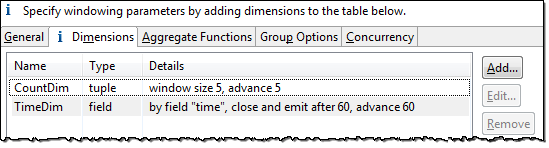Open the Edit Dimension dialog in one of the following ways. In the Properties view for an Aggregate operator, in the Dimensions tab:

• Double-click an existing dimension row.

• Select an existing dimension row and click .

• Click .

Then, in the resulting Edit Dimension dialog, select one of the values in the Type drop-down list: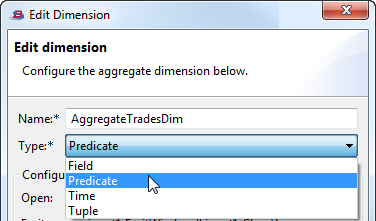The dialog then reconfigures itself to conform to the selected dimension type. The options are:

• Field — windows are managed based on input tuple field values.

• Predicate — windows are managed based on predicate expressions for opening, emitting from, and closing the window.

• Time — windows are managed based on the passage of time.

• Tuple — windows are managed based on the number of input tuples.

### Important

TIBCO recommends avoiding the time-based dimension for most applications. See the Caution on Aggregate Operator: Time-Based Dimension Options for an explanation and suggested alternatives.

## Properties: Aggregate Functions Tab

Use the Aggregate Functions tab to specify one or more aggregate expressions that will be evaluated in each window. The result of each specified aggregate function is added as a field to the operator's output stream. You can add, replace, or remove fields from appearing in the output stream.

For a new Aggregate operator newly placed on the EventFlow canvas, the grid in this tab has a single entry with the following default values:

Action Field Name Expression

In the example below, the first expression calculates the average of prices in the window. The next two expressions capture the start and end time of the window (the argument indicates a time-based dimension).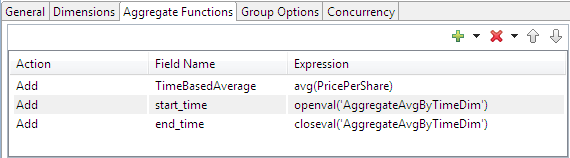The Aggregate Functions grid has the following editing features:

• The grid is resizable. Grab the bottom row with the mouse to resize it within the Aggregate Functions tab to show fewer rows or to show more rows without scroll bars.

• To add a grid row, use the green plus button, then select the type of action in the Action drop-down list.

• Available Action column entries for each grid row are: Add, Replace, Remove, and Declare.

• When using the Remove action, you must specify a field name, but you cannot enter an expression.

• When using the Add and Replace actions, you must specify a field name, and you must enter an expression in the Expression field for that row.

• Use the Declare action to define a local variable that has the narrow scope of this grid in this operator. The variable can then be used to save typing in expressions later in the same grid. Use the Field Name column to name your variable; use the Expression column to specify an expression that defines your variable. Expressions for declared variables are evaluated as necessary to compute the output fields that use the variable. In many cases expressions are evaluated once per output tuple. However, expressions for unused declared variables are not evaluated. You can declare variables for aggregate expressions as well as simple expressions.

• Expressions can contain aggregate functions and constants. Besides field names that are part of aggregate function calls, direct references to input stream fields must be grouped, as described in Properties: Group Options Tab.

• For a list of aggregate functions, see StreamBase Expression Language Functions.

The following table describes the buttons at the top of the Field grids.

Button Name Description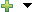Add Adds a row below the currently selected row, or to the end of the grid if none are selected. Click the arrow on the button's right to specify whether the row should be added above or below the currently selected row.

When you add a row, the newly created row is highlighted. To start entering information, click in the cell you want to edit. (Some cells are not user-editable.)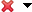Remove Removes the currently selected row. Click the arrow on the button's right to remove all rows or all selected rows.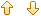Move Up, Move Down moves the selected row up by one row. moves the selected row down by one row.

## Properties: Group Options Tab

In general, the Aggregate operator controls aggregate windows according the dimension properties you define. In addition, the Group Options tab allows you to logically partition the stream according to groupings of input tuple field values that you define. For example, if you create a Group By field named `ID` whose Expression property is `input1.ID`, then there a separate window is opened for each unique value of the `input1.ID` field that the Aggregate operator processes. If you have multiple Group By fields, there a separate window is created for each unique combination of values of all of the Group By fields.

Click the button to add a row for each group-by field that you want to define. In each row:

• In the Output Field Name column, enter the name of the field as you want it to appear in this operator's output schema.

• In the Expression column, enter the aggregate expression that you want to group on.

By default, all Group By fields appear in the schema of the tuples output by the Aggregate operator that defines them. You can suppress appending any Group By fields to the output tuple by selecting the check box labeled Omit Group By fields in output.

In the following example, the Aggregate operator generates separate windows according to the operator's dimension properties for each of the unique processed values of the `Symbol` field, and the resulting field in the output tuple is named `TotalForSymbol`.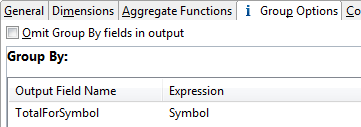## Properties: Concurrency Tab

Use the Concurrency tab to specify parallel regions for this instance of this component, or multiplicity options, or both. The Concurrency tab settings are described in Concurrency Options, and dispatch styles are described in Dispatch Styles.

### Caution

Concurrency settings are not suitable for every application, and using these settings requires a thorough analysis of your application. For details, see Execution Order and Concurrency, which includes important guidelines for using the concurrency options.

## Null Values

In general, in an Aggregate operator window, null field values are not included in the calculation. For example: in an average, such as `avg(price)` of ten tuples in an aggregate's window, if one of the values is null, then the average is calculated for the remaining nine values. Null handling for the andall() and orall() functions does not follow this general rule.

Independent of the preceding paragraph, key fields with null values still constitute a valid group for the purposes of grouping aggregate results. For example, let's say a simple Aggregate operator averages prices for every three tuples with schema `{symbol, price}`, and groups the results by symbol. If three tuples arrive with price information but an empty `symbol` field, the operator still reports an average of those three for the `null` group.

## Example 1: Simple Aggregate Operation

This example demonstrates the basic Aggregate operation by tracing the windowing action in a simple application with these characteristics:

• Each tuple in the input stream is based on a schema that contains a single integer field.

• The Aggregate operator has a single tuple-based dimension. The dimension is set to advance each window by one tuple, and each window is set to close and emit after three tuples. There are no group-by settings.

• The `sum()` aggregating function calculates the total value of all integers in each window.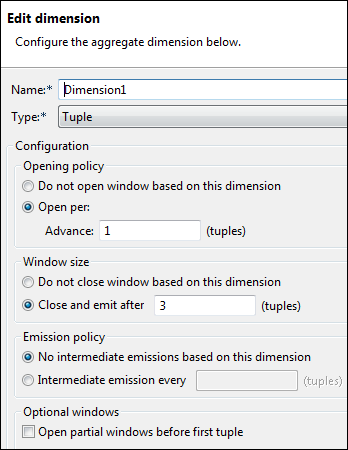The following diagrams show a succession of tuples arriving on an input stream. At each step, we depict the arrival of a tuple, the windows that are open at that time, the aggregate function performed on each window, and any output that occurs.

1. When the first tuple arrives on the input stream, a window is immediately opened. Consider the following points:

• The new window contains the data for the first tuple, but it has room for three tuples, since the dimension sets the window size to `3`.

• The diagram shows the aggregation function that is performed on the window (even though here it is only a sum of one number). As more data is enqueued, the window keeps a running sum of tuple values.

• Because the window is not full, no output occurs. Remember that the dimension is set to emit when the size is `3`.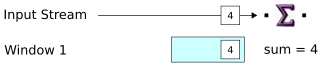2. When a new tuple arrives, a second window is opened and the new tuple is loaded in both windows. This is in accordance with the dimension, which sets windows to advance by one tuple. If the advance had been set to `2`, a new window would open every two tuples (in the next step) instead.

Notice the positions of the two windows relative to the tuples in the input stream. For readability, the diagram represents each window separately. In fact, both windows operate on the same data. A more realistic representation might show a succession of overlapping windows moving over a single stream of data.

Also notice that the aggregate result changes for Window 1, and that there is still no output from the operator.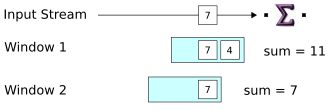3. When the third tuple arrives, a new window is created and all the windows are updated. For the first time, we see a tuple released on the operator's output port. The output tuple contains the aggregate result from Window 1, which is now full. As soon as the output tuple is emitted, the Aggregate operator closes the window.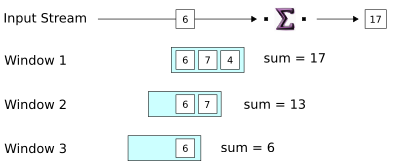4. When the fourth tuple arrives, a new window is started, as before. Notice that Window 1, which we said was closed in the previous step, is gone now. At the same time, the new tuple causes Window 2 to reach its size limit. This triggers the emission of Window 2's aggregate result in an output tuple, and Window 2 is closed.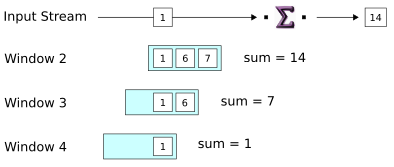5. The last step shown is just like the preceding one: input causes a window to become full and release its aggregate result on the output port. And again, a new window opens one tuple ahead of the preceding window,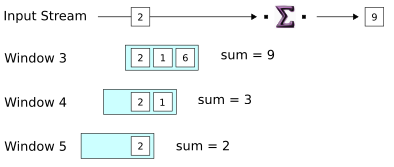## Example 2: Counting Tuples

This example demonstrates a dimension and related Aggregate function that generate a sequential count of received tuples on the output stream. In current StreamBase releases, the same functionality would be better handled with the Sequence operator, but this example is nevertheless instructive.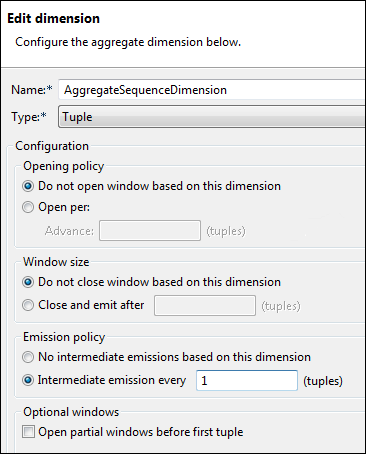In this example, notice that we selected these two options:

• Opening policy: Do not open window based on this dimension

• Window size: Do not close window based on this dimension

That combination of settings creates a window that never closes (while StreamBase Server is running, of course). Also notice the setting of the Emission policy, which gives you the option of emitting output before the window closes. Here, the policy is set to Intermediate emission every 1 tuple. This means that, as every tuple arrives, one tuple is emitted.

In the Aggregating Functions tab, the operator is set to use the `count()` aggregate function: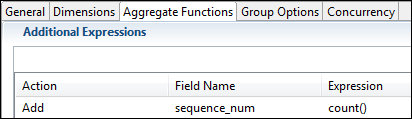As each tuple arrives in the Aggregate operator, it is counted. Because each tuple causes a tuple to be emitted, the emitted tuple contains a value in the `sequence_num` field that is one greater than the previous tuple.

To understand how the `count()` function is used here, and for further information on aggregate functions in general, see the Aggregate Functions Overview section of the Expression Language Functions topic in the StreamBase References.

Look for further examples of using the Aggregate operator in the Operator Sample Group provided with your StreamBase installation.